Science, Maths & Technology

### Become an OU student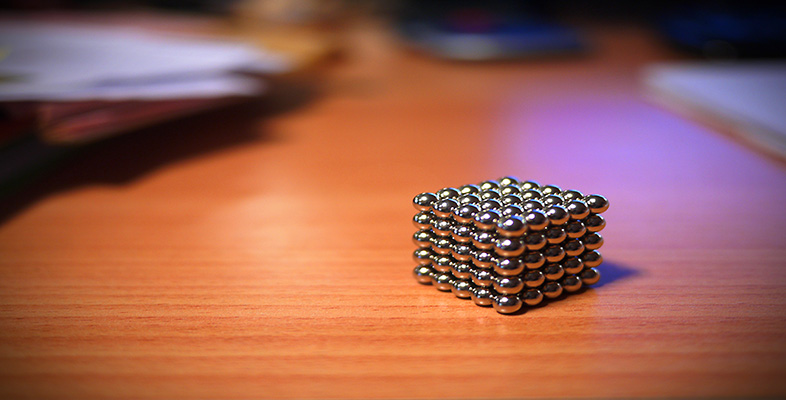Squares, roots and powers

Start this free course now. Just create an account and sign in. Enrol and complete the course for a free statement of participation or digital badge if available.

# 1.3.1 Try some yourself

## Activity 6

The new home owners from Example 4 above want to price grass seed, as well as the turf (transport only). The best buy seems to be loose seed, which says ‘1 kilo covers 80 m2’. They wonder what length the side of an 80 m2 square lawn would be? Make an estimate and then use your calculator to find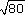and hence the side of the square lawn.

A square of side 9 m has an area of 81 m2. So 80 m2 would be a little less than this.= 8.94.... So the length of the side of the square lawn would be 8.9 m.

## Activity 7

Use your calculator to find each of the following, estimating the answer first in each case.

• (a)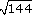• (b)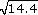• (c)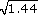• (d)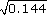• (a) Since 12 × 12 = 144, the answer must be= 12. The calculator confirms this.

• (b) Since 32 = 9 and 42 = 16,must lie between 3 and 4.

• Calculator gives3.7947 rounded to four decimal places.

• (c) Since 12 = 1 and 22 = 4,must lie between 1 and 2.

Calculator gives= 1.2.

• (d) Since 0.32 = 0.09 and 0.42 = 0.16,must lie between 0.3 and 0.4.

Looking at the pattern of parts (a) and (c), and since= 3.7947, a good guess would be that= 0.3795 rounded to four decimal places.

Indeed, the calculator gives0.3795 rounded to four decimal places.